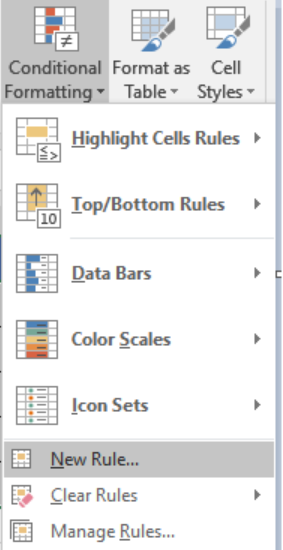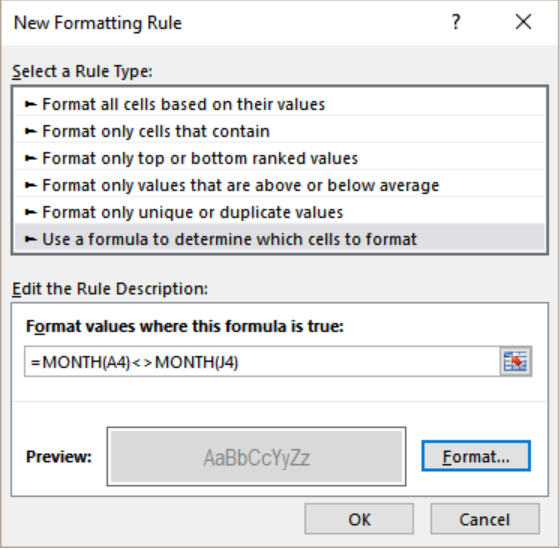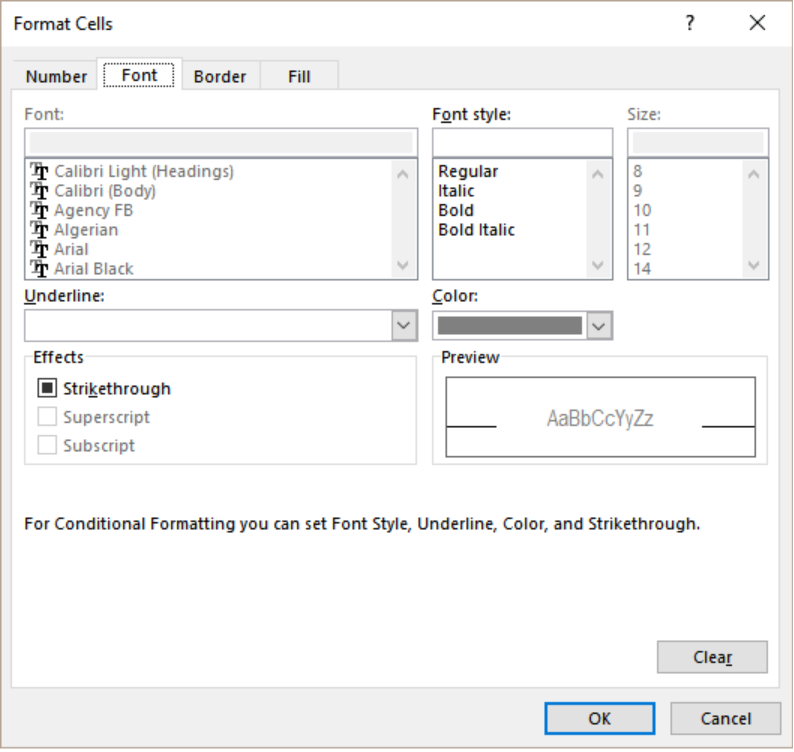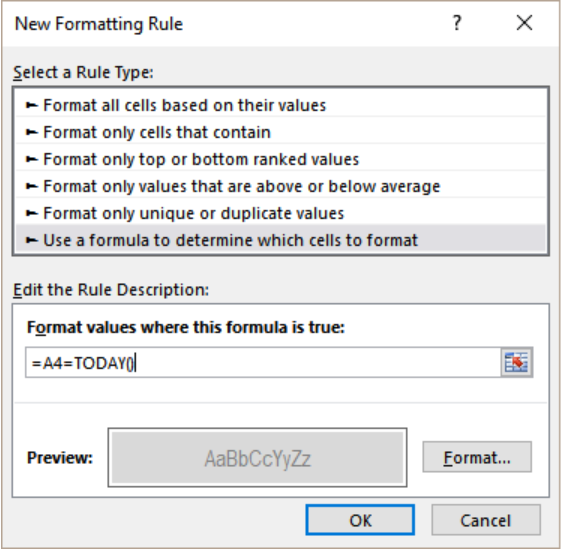Get instant live expert help with Excel or Google Sheets“My Excelchat expert helped me in less than 20 minutes, saving me what would have been 5 hours of work!”

#### Post your problem and you’ll get expert help in seconds.

Your message must be at least 40 characters
Our professional experts are available now. Your privacy is guaranteed.

# Dynamic calendar grid

Excel offers some very helpful features to work with dates. One of these is the dynamic calendar grid. We can have a start date that is the start of the month and create a dynamic calendar grid. The WEEKDAY and CHOOSE functions with conditional formatting help us to create this. In this tutorial, we will learn how to create a dynamic calendar grid in Excel.Figure 1. Example of How to Use the Dynamic Calendar Grid in Excel

## Generic Formula

`=start-CHOOSE(WEEKDAY(start),0,1,2,3,4,5,6)`

## Process

Here, we are using the CHOOSE and WEEKDAY functions. This formula calculates the Sunday before the first day of the month. It does so by using the CHOOSE function. It rolls back to the right number of days to the previous Sunday. CHOOSE allows random values for each day of the week. This feature rolls back zero days if the first day of the month is a Sunday. It adjusts the roll back for the rest of the days.

After the first day is extracted, the other formulas in the grid adds one to the previous date. It checks the cell on the left for a value. If it does not find a value, it pulls a value from the rightmost column in the row above. We assign this value as a mixed reference. We do this to ensure the column is locked as the formula is copied throughout the grid. The same formula is used in all other cells.

## Setting up Data

The following example contains a start date. It is in cell J5. From this value, we will create a dynamic calendar grid in column A to G.

To do that, we need to:

• Go to the View tab on the ribbon. Uncheck Gridlines.
• Next, we need to select cells A3 to G9. Go to the Home tab on the ribbon. Click on Borders>All borders.
• Click on cell A2. Assign the formula =J6.
• Right click on A2. Select Format Cells>Numbers>Custom. On the Type box type mmmm yyyy. Click Ok.
• Select A2 to G2. From the Home tab select Merge and Center.
• Enter the weekday abbreviations in cells A3 to G3.
• Go to cell A4. Apply the formula `=``J6-CHOOSE(WEEKDAY(J6),0,1,2,3,4,5,6)`
• Select cells A4 to G8. Right click and select Format Cells>Numbers>Custom. On the Type box type d. Click Ok.
• Go to cell B4. Apply the formula `=IF(A4<>"",A4,\$J6)+1`. Drag it to the remaining cells in the calendar grid.
• Select cells A4 to G8. From the home tab in the ribbon, go to Conditional Formatting. Next, we need to click on New Rule.Figure 2. Example of how to Apply Conditional Formatting

• Select Use a formula to determine which cells to format.
• Click the Format values where this formula is true box. On the formula box, assign the formula `=MONTH(A4)<>MONTH(J4)`.Figure 3. Applying the Formula to the Conditional Format

• Click the Format tab near the preview box.
• Next, we need to go to  Fill>Background Color and select the color we want to highlight in.Figure 4. Managing the Display Options

• Click OK twice.
• To format the current date follow steps 10 to 14. Change the formula to `=A4=TODAY()`.Figure 5. Applying the format to Current Date

## Notes

We can create a calendar that updates automatically based on the current date. To do that we need to use the formula =EOMONTH(TODAY(),-1)+1 in J6. This formula fetches the current date with the TODAY function. Later, it gets the first day of the running month using EOMONTH. By replacing TODAY() with any other date, we can build a calendar in another month.

Most of the time, the problem you will need to solve will be more complex than a simple application of a formula or function. If you want to save hours of research and frustration, try our live Excelchat service! Our Excel Experts are available 24/7 to answer any Excel question you may have. We guarantee a connection within 30 seconds and a customized solution within 20 minutes.

### Did this post not answer your question? Get a solution from connecting with the expert.Another blog reader asked this question today on Excelchat:
Solution examplesI would need a formula what copies from my DataInput sheet into another one daily the first two column A and B.
Solved by K. S. in 33 minsI would need a formula what copies from my DataInput sheet into another one daily the first two column A and B.
Solved by B. C. in 60 minsI need the formula for time accrual to auto populate in E4 based on certain criteria. example: B4 = hire date E4= accrual rate If todays date is equal to or greater than 60 days but less than 1 year from B4 date then E4 = 1 If todays date is equal to or greater than 3 years but less than 4 years from B4 date then E4 = 1.08 If todays date is equal to or greater than 5 years but less than 9 years from B4 date then E4 = 1.5 If todays date is equal to or greater than 10 years but less than 14 years from B4 date then E4 = 1.58 If todays date is equal to or greater than 15 years from B4 date then E4 = 1.92
Solved by A. H. in 60 minsHello. I would like to create a drop down option to choose Square Feet or Square Meters. Next column they insert the amount and the next it converts the value to whatever is chosen from the drop down option. Can you help please?
Solved by Z. Q. in 39 minsHi there. I need a column to automatically calculate the numbers of days from a start time to today's date.
Solved by T. F. in 16 mins## Subscribe to Excelchat.coAnother blog reader asked this question today on Excelchat: